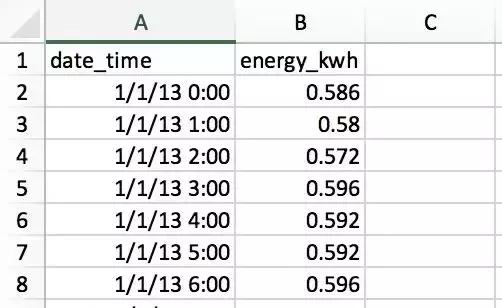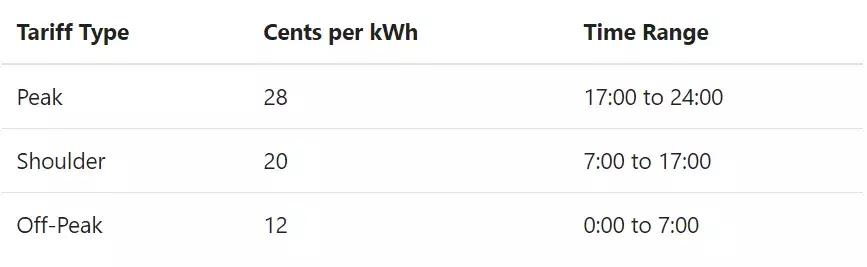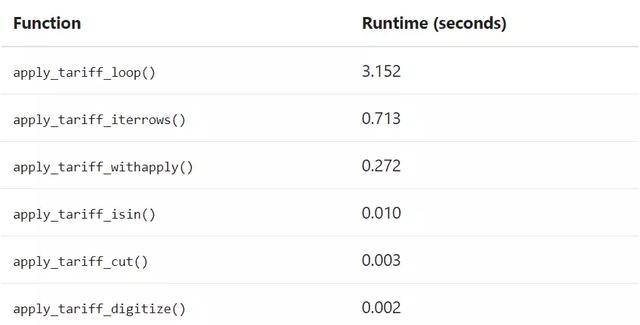python使用Pandas库提升项目的运行速度过程详解

更新时间：2019年07月12日 10:51:46   作者：千锋Python唐唐君我要评论

“快速、灵活、和易于理解的数据结构，以此让处理关系型数据和带有标签的数据时更简单直观。”

• ·使用datetime时间序列数据的优势
• ·处理批量计算更效率的方法
• ·利用HDFStore节省时间

【注】

【工具】

Python 3、Pandas 0.23.1>>z import pandas as pd
>>> pd.__version__
'0.23.1'
>>> df = pd.read_csv('文件路径')
date_time energy_kwh
0 1/1/13 0:00 0.586
1 1/1/13 1:00 0.580
2 1/1/13 2:00 0.572
3 1/1/13 3:00 0.596
4 1/1/13 4:00 0.592

>>> df.dtypes
date_time object
energy_kwh float64
dtype: object
>>> type(df.iat[0, 0])
str

>>> df['date_time'] = pd.to_datetime(df['date_time'])
>>> df['date_time'].dtype
datetime64[ns]

date_time energy_kwh
0 2013-01-01 00:00:00 0.586
1 2013-01-01 01:00:00 0.580
2 2013-01-01 02:00:00 0.572
3 2013-01-01 03:00:00 0.596
4 2013-01-01 04:00:00 0.592

>>> @timeit(repeat=3, number=10)
... def convert(df, column_name):
... return pd.to_datetime(df[column_name])
>>> # Read in again so that we have `object` dtype to start
>>> df['date_time'] = convert(df, 'date_time')
Best of 3 trials with 10 function calls per trial:
Function `convert` ran in average of 1.610 seconds.

>>> @timeit(repeat=3, number=100)
>>> def convert_with_format(df, column_name):
... return pd.to_datetime(df[column_name],
... format='%d/%m/%y %H:%M')
Best of 3 trials with 100 function calls per trial:
Function `convert_with_format` ran in average of 0.032 seconds.>>> df['cost_cents'] = df['energy_kwh'] * 28

date_time energy_kwh cost_cents
0 2013-01-01 00:00:00 0.586 16.408
1 2013-01-01 01:00:00 0.580 16.240
2 2013-01-01 02:00:00 0.572 16.016
3 2013-01-01 03:00:00 0.596 16.688
4 2013-01-01 04:00:00 0.592 16.576
# ...

def apply_tariff(kwh, hour):
"""电价函数"""
if 0 <= hour < 7:
rate = 12
elif 7 <= hour < 17:
rate = 20
elif 17 <= hour < 24:
rate = 28
else:
raise ValueError(f'Invalid hour: {hour}')
return rate * kwh

>>> # 注意：不要尝试该函数！
>>> @timeit(repeat=3, number=100)
... def apply_tariff_loop(df):
... """用遍历计算成本，将结果并入到df中"""
... energy_cost_list = []
... for i in range(len(df)):
... # 获取每个小时的耗电量
... energy_used = df.iloc[i]['energy_kwh']
... hour = df.iloc[i]['date_time'].hour
... energy_cost = apply_tariff(energy_used, hour)
... energy_cost_list.append(energy_cost)
... df['cost_cents'] = energy_cost_list
...
>>> apply_tariff_loop(df)
Best of 3 trials with 100 function calls per trial:
Function `apply_tariff_loop` ran in average of 3.152 seconds.

Pandas实际上通过引入DataFrame.itertuples()和DataFrame.iterrows()方法使得for i in range(len(df))语法冗余。这两种都是产生一次一行的生成器方法。

.itertuples()为每行生成一个nametuple类，行的索引值作为nametuple类的第一个元素。nametuple是来自Python的collections模块的数据结构，该结构和Python中的元组类似，但是可以通过属性查找可访问字段。

.iterrows()为DataFrame的每行生成一组由索引和序列组成的元组。

>>> @timeit(repeat=3, number=100)
... def apply_tariff_iterrows(df):
... energy_cost_list = []
... for index, row in df.iterrows():
... #获取每个小时的耗电量
... energy_used = row['energy_kwh']
... hour = row['date_time'].hour
... # 增加成本数据到list列表
... energy_cost = apply_tariff(energy_used, hour)
... energy_cost_list.append(energy_cost)
... df['cost_cents'] = energy_cost_list
...
>>> apply_tariff_iterrows(df)
Best of 3 trials with 100 function calls per trial:
Function `apply_tariff_iterrows` ran in average of 0.713 seconds.

Pandas的.apply()

>>> @timeit(repeat=3, number=100)
... def apply_tariff_withapply(df):
... df['cost_cents'] = df.apply(
... lambda row: apply_tariff(
... kwh=row['energy_kwh'],
... hour=row['date_time'].hour),
... axis=1)
...
>>> apply_tariff_withapply(df)
Best of 3 trials with 100 function calls per trial:
Function `apply_tariff_withapply` ran in average of 0.272 seconds.

.apply()的语法优势很明显，代码行数少了，同时代码也更易读了。运行速度方面，这与.iterrows()方法相比节省了大约一半时间。

df.set_index('date_time', inplace=True)
@timeit(repeat=3, number=100)
def apply_tariff_isin(df):
# 定义每个时段的布尔型数组(Boolean)
peak_hours = df.index.hour.isin(range(17, 24))
shoulder_hours = df.index.hour.isin(range(7, 17))
off_peak_hours = df.index.hour.isin(range(0, 7))
# 计算不同时段的电费
df.loc[peak_hours, 'cost_cents'] = df.loc[peak_hours, 'energy_kwh'] * 28
df.loc[shoulder_hours,'cost_cents'] = df.loc[shoulder_hours, 'energy_kwh'] * 20
df.loc[off_peak_hours,'cost_cents'] = df.loc[off_peak_hours, 'energy_kwh'] * 12

>>> apply_tariff_isin(df)
Best of 3 trials with 100 function calls per trial:
Function `apply_tariff_isin` ran in average of 0.010 seconds.

[False, False, False, ..., True, True, True]

@timeit(repeat=3, number=100)
def apply_tariff_cut(df):
cents_per_kwh = pd.cut(x=df.index.hour,
bins=[0, 7, 17, 24],
include_lowest=True,
labels=[12, 20, 28]).astype(int)
df['cost_cents'] = cents_per_kwh * df['energy_kwh']

pd.cut()根据分组bins产生的区间生成对应的标签“费率”。

【注】include_lowest参数设定第一个间隔是否包含在组bins中，例如想要在该组中包含时间在0时点的数据。

>>> apply_tariff_cut(df)
Best of 3 trials with 100 function calls per trial:
Function `apply_tariff_cut` ran in average of 0.003 seconds.

@timeit(repeat=3, number=100)
def apply_tariff_digitize(df):
prices = np.array([12, 20, 28])
bins = np.digitize(df.index.hour.values, bins=[7, 17, 24])
df['cost_cents'] = prices[bins] * df['energy_kwh'].values

>>> apply_tariff_digitize(df)
Best of 3 trials with 100 function calls per trial:
Function `apply_tariff_digitize` ran in average of 0.002 seconds.

Pandas可以提供很多批量处理数据方法的备用选项，这些已经在上边都一一演示过了。这里将最快到最慢的方法排序如下：

1. 使用向量化操作：没有for遍历的Pandas方法和函数。

2. 使用.apply()方法。

3. 使用.itertuples()：将DataFrame行作为nametuple类从Python的collections模块中进行迭代。

4. 使用.iterrows()：将DataFrame行作为（index，pd.Series）元组数组进行迭代。虽然Pandas的Series是一种灵活的数据结构，但将每一行生成一个Series并且访问它，仍然是一个比较大的开销。

5. 对逐个元素进行循环，使用df.loc或者df.iloc对每个单元格或者行进行处理。

【注】以上顺序不是我的建议，而是Pandas核心开发人员给的建议。Pandas有个内置的解决方案，它使用HDF5，这是一种专门用于存储数组的高性能存储格式。Pandas的HDFstore方法可以将DataFrame存储在HDF5文件中，可以有效读写，同时仍然保留DataFrame各列的数据类型和其他元数据。它是一个类似字典的类，因此可以像Python中的dict类一样读写。

# 创建存储类文件并命名 `processed_data`
data_store = pd.HDFStore('processed_data.h5')
#将DataFrame写入存储文件中，并设置键（key） 'preprocessed_df'
data_store['preprocessed_df'] = df
data_store.close()

# 访问数据仓库
data_store = pd.HDFStore('processed_data.h5')
# 读取键（key）为'preprocessed_df'的DataFrame
preprocessed_df = data_store['preprocessed_df']
data_store.close()

【注】使用Pandas的HDFStore需要安装PyTables>=3.0.0，因此安装Pandas后，需要更新PyTables：

pip install --upgrade tables

① 尝试更多的向量化操作，尽量避免类似for x in df的操作。如果代码中本身就有许多for循环，那么尽量使用Python自带的数据结构，因为Pandas会带来很多开销。

② 如果因为算法复杂无法使用向量化操作，可以尝试.apply()方法。

③ 如果必须循环遍历数组，可用.iterrows()或者.itertuples()改进语法和提升速度。

④ Pandas有很多可选项操作，总有几种方法可以完成从A到B的过程，比较不同方法的执行方式，选择最适合项目的一种。

⑤ 做好数据处理脚本后，可以将中间输出的预处理数据保存在HDFStore中，避免重新处理数据。

⑥ 在Pandas项目中，利用NumPy可以提高速度同时简化语法。